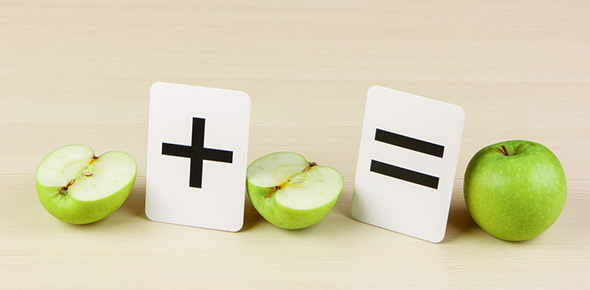# Fraction Action

7 Questions | Total Attempts: 35SettingsThis Fraction quiz is a phenomenal way to get from 0 to Hero. At first it is basic then it gets massively hard! TAKE FRACTION ACTION TODAY! t IF YOU SCORE ABOVE 80% YOU PASS AND GET A CERTIFICATE

Related Topics
• 1.
Write a example of how to divide and write the process of dividing fractions.
• 2.
FREE QUESTIONMake a fraction equation and solve it.Extra challenge:Tell how you did it.
• 3.
Give 5 ways to write 6/12.You can describe as well.BIG BOMB BONUS: For a score of 1million! Give 9 reasons why you should learn fractions if your becoming a company worker.
• 4.
What is "18" in this fraction 18/99?
• A.

2/11

• B.

Denominator

• C.

Equivalent Fraction

• D.

Whole Number

• E.

None Of The Above

• 5.
Name 5 other Equivalent fractions in order to 1/3. Note: Don't list 1/3. Put a comma space after each fraction.Hint: Multiply 2 to the numerator and denominator to each previous answer to get the next.
• 6.
In this algebraic problem what does X represent? (9/14 + 5/6) - (9/89 / 7/4) + X = 234 All the others were practice here comes TEST!
• A.

C= 232 1086/1867

• B.

X = 232 1092/1400

• C.

X = 232 1087/1869

• D.

X = 231 1098/5652

• E.

None of the above

• 7.
Josh my friend kicked a soccer ball 900 1/4 inches. I kicked my soccer ball 1/4 times joshes. Jaylen kicked 4/16 times Joshes kick. What statements are correct below? Check as many that follow.
• A.

I kicked 226 centimeters.

• B.

Me and Jaylen are tied.

• C.

Josh kicked farthest

• D.

None of the above

• E.

All of the above.